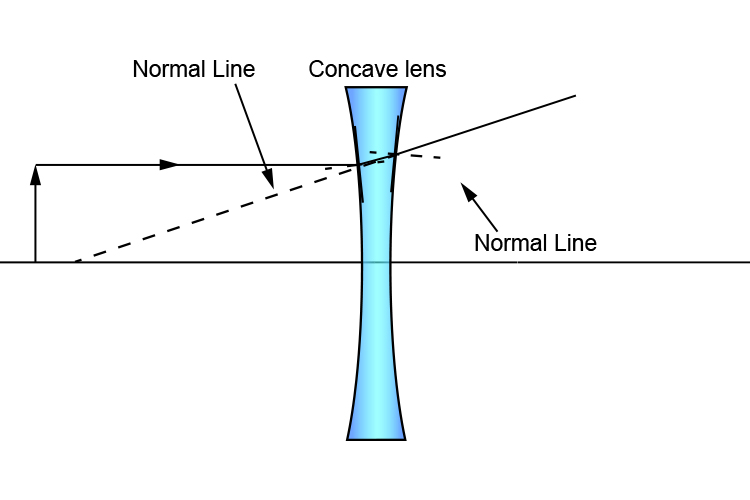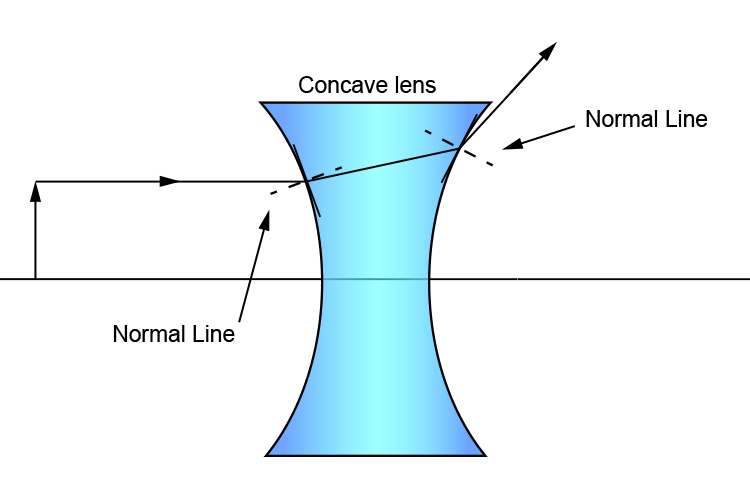# Concave lens – Principal focal length and centre of curvature

If you have two identically shaped concave lenses but they are made of different materials, parallel rays of light on each will have different principal focal lengths.

However, two concave lenses made out of the same material (same refractive index (n)) will have different principal focal lengths if they have different centres of curvature (radius of spheres). Another way of saying this is, if two lenses of the same material have different shapes – one a slightly curved surface and the other a rounded one – then they will have different principal focal lengths.

Slightly curved lens = Long focal length
Very curved lens = Short focal length

This should be easy to remember because the ray of light passing through the lens is affected by how curved the lens is.

As you can see below, the ray's line hitting the large centre of curvature (slightly curved lens) doesn't deviate from the normal line as much as the ray hitting the smaller centre of curvature (very curved lens).

Large centre of curvature (slightly curved lens):Small centre of curvature (very curved lens):